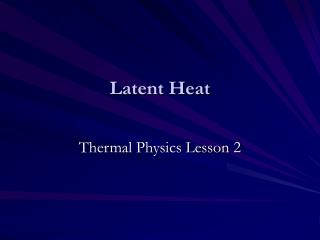DownloadDownload PresentationLatent Heat

# Latent Heat

Télécharger la présentation## Latent Heat

- - - - - - - - - - - - - - - - - - - - - - - - - - - E N D - - - - - - - - - - - - - - - - - - - - - - - - - - -
##### Presentation Transcript

1. Latent Heat Thermal Physics Lesson 2

2. Learning Objectives • Define specific latent heat. • Perform calculations using ∆Q=ml. • Describe how specific latent heat of fusion/vaporisation can be measured in the lab. • Explain why energy is needed to evaporate a liquid/melt a solid

3. Safety • Students must not sit down to watch this experiment - serious scalding has occurred when the beaker breaks or falls and the pupil has been unable to move away instantly.

4. Heating/Cooling Curves Remember from GCSE?

5. Demonstration • What tells you the water is boiling? • So energy is being supplied but the temperature is not rising? • What is going on? • Work is being done to separate the particles against intermolecular attractive forces.

6. shc vs. latent heat (simple terms) Specific Heat Capacity:- • Energy needed to heat something Latent Heat:- • Energy needed to change phase

7. Definition • The specific latent heat (l) of fusion or vaporisation is the quantity of thermal energy required to change the phase of 1kg of a substance. • Fusion (liquid  solid) • Vaporisation (liquid  gas) • …or the other way (Melting/Condensing)

8. Equation where:- ∆Q is the energy change in J m is the mass of substance changing phase in kg lv is the latent heat of vaporisation in J kg-1 lf is the latent heat of fusion in J kg-1

9. Worked Example 1 • The specific latent heat of fusion (melting) of ice is 330,000 J kg-1. What is the energy needed to melt 0.65 kg of ice?

10. Worked Example 1 • The specific latent heat of fusion (melting) of ice is 330,000 J kg-1. What is the energy needed to melt 0.65 kg of ice? • ∆Q = ml = 0.65 kg × 330,000 J kg-1 = 210,000 J (2 s.f.)

11. (resourcefulphysics.org) Worked Example 2 • The power of the immersion heater in the diagram is 60 W. In 5 minutes, the top pan balance reading falls from 282g to 274g. What is the specific latent heat of vaporisation of water?

12. (resourcefulphysics.org) Worked Example 2 • The power of the immersion heater in the diagram is 60 W. In 5 minutes, the top pan balance reading falls from 282g to 274g. What is the specific latent heat of vaporisation of water? • P = 60 W • ∆ t = 5 minutes = (5 × 60)s = 300 s • m = m2 -m1 = 282g – 274g = 8g = 0.008 kg • lv = ? • ∆Q = P∆ t = 60 W × 300s= 18,000 J • lv = ∆Q/m = 18,000 J / 0.008 kg= 2.3 × 106 J kg-1 (2 s.f.)